# Data Analysis

## Introduction to Recommender Systems1

1. Some materials are taken from machine learning course of Victor Kitov

## Motivation¶

• Everyday people have to make decisions:

• what music to listen
• what movies to see
• what books to read
• what food to eat
• what games to play
• ...
• Multitude of choices

• Spotify - 30kk. songs
• Netflix - 20k. movies and series
• Amazon - 500k. books
• Steam - 20k games

To filter out the seach people can rely on recommendations

• of their friends (if there are friends)
• of some reviews

These recommendations are too narrow and standard

• rely on information of a handful of people
• not tuned for individual user tastes

Need automatic recommendation system!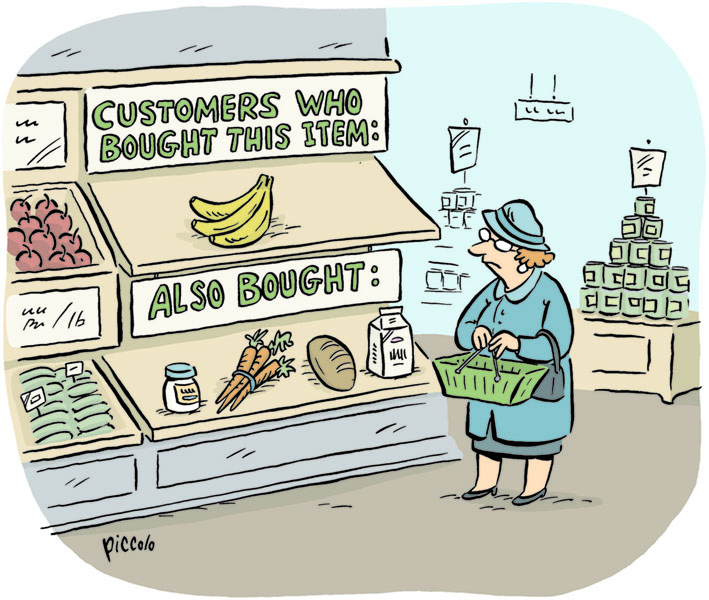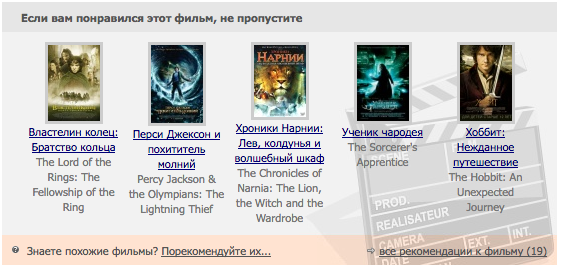### Long Tail Phenomenon¶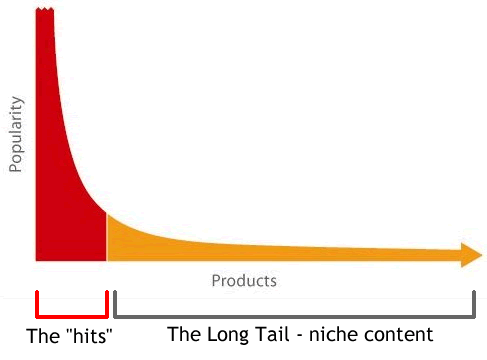# Few words on history¶

## Netflix prize¶

• Competition for the best recommendation algirithm
• October 2007 - September 2009
• Prize: 1 000 000 $• 480 000 users • 17 770 movies • format: [user, movie, datetime, rating] • grades: 1,2,3,4,5 • quality measure: RMSE ### Netflix Prize¶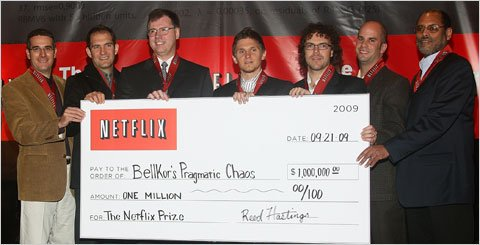### Recsys¶ Annual international conference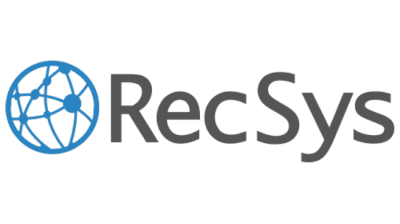# Collaborative filtering¶ ## Collaborative filtering¶ A method of making automatic predictions about the interests of a user by collecting prefences or taste information from similar users • Generic CF algorithm 1. Look for users who share the same rating patterns with current user 2. Use their ratings to predict currently unknown user ratings • Main assumption: if person A has the same opinion as a persion B on an issue, A is more likely to have B's opinion on a different issue. • Have you already seen this idea? ## Collaborative filtering¶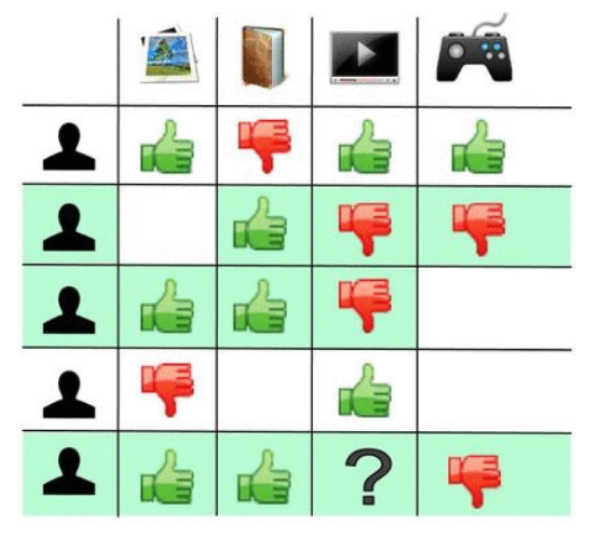## Challenges¶ • Scalability • many users and items • Cold start • new user has not rated many items - hard to predict his preferences • new item was not rated by many users - hard to predict its characteristics • Shilling attacks • shop representatives may give lots of positive ratings for their own items ang negative ratings for theis competitors • Learning Loop • once the model have been relearned it may relearned its previous predictions - does not gain new knowledge • Obvious recommendations # Core concepts¶ • Users give ratings to items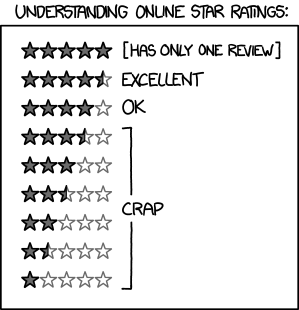• Users give ratings to items • Binary • "Stars" • Implicit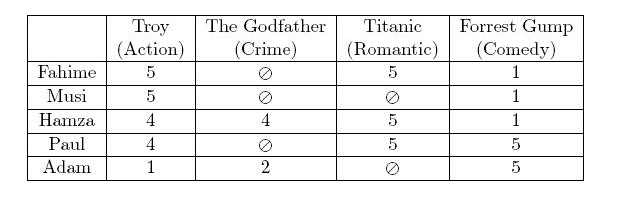• Tasks of RecSys: • Fill missing values of the ratings • Recommend items to user • may not be the highest ranked! ## Notations¶ •$U$- set of all users •$I$- set of all items •$u$,$v$- some users •$i$,$j$- some items •$U_i$- set of users, that rated item$i$•$I_u$- set of items, that have been rated by user$u$•$R_{ui}$- actual rating of item$i$by user$u$•$\hat{R}_{ui}$- predicted rating of item$i$by user$u$# Baseline¶ ## Baseline ideas¶ •$b_{ui} = \mu$,$(\mu = \frac{1}{n}\sum\limits_{u,i} R_{ui})$•$b_{ui} = \bar{R}_u = \frac{1}{|I_u|}\sum\limits_{i\in I_u} R_{ui}$•$b_{ui} = \bar{R}_i = \frac{1}{|U_i|}\sum\limits_{u\in U_i} R_{ui}$## General baseline¶ •$b_{ui} = \mu + b_u + b_i$, •$b_{u} = \frac{1}{|I_u|}\sum_{i\in I_u}(R_{ui} - \mu)$•$b_{i} = \frac{1}{|U_i|}\sum_{u\in U_i}(R_{ui} - b_u - \mu)$Intuition •$b_u$is how much higher user rates items than on average •$b_i$is how much item$i$is rated higher than average user rating ## General baseline¶ •$b_{ui} = \mu + b_u + b_i$, •$b_{u} = \frac{1}{|I_u|+\alpha}\sum_{i\in I_u}(R_{ui} - \mu)$•$b_{i} = \frac{1}{|U_i|+\beta}\sum_{u\in U_i}(R_{ui} - b_u - \mu)$• What is the idea behind$\alpha$and$\beta$? ## Motivation¶ • Compare accuracy with advanced model • Can compute missing values with baseline values • Normalization: predict$R_{ui} - b_{ui}$instead of$R_{ui}$# User-based Collaborative filteting¶ ## User-based CF¶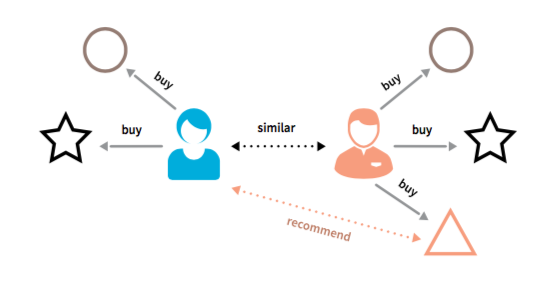## User-based CF¶ • Also known as K-NN CF • Define similarity function on users:$s \in \mathbb{R}^{U \times U}$• Algorithm with level normalization: 1. For user$u$find a neighbourhood of similar users$N(u)$2. Generate rating predictions by averaging over$N(u)$$$\hat{R}_{ui} = \bar{R}_u + \frac{\sum_{v \in N(u)} s_{uv}(R_{vi} - \bar{R}_v)}{\sum_{v \in N(u)} \left| s_{uv}\right|}$$ •$\bar{R}_u$- adjustment to compensate average rating behaviour of u • skeptics/optimists normalization ## User-based CF¶ • Algorithm with level and scale normalization: 1. For user$u$find a neighbourhood of similar users$N(u)$2. Generate rating predictions by averaging over$N(u)$$$\hat{R}_{ui} = \bar{R}_u + \sigma_u\frac{\sum_{v \in N(u)} s_{uv}(R_{vi} - \bar{R}_v)/\sigma_v}{\sum_{v \in N(u)} \left| s_{uv}\right|}$$ • where$\sigma_u$is standard deviation of user$u$ratings ## Number of NN¶ • In general 20-50 is suggested • Also possible • Take all • Top-k •$s_{uv} > \theta$## Similarity measures¶ • For each pair$(u,v)$the intersection of rated items should be considered! • Pearson correlation $$s_{uv} = \frac{\sum\limits_{i \in I_u\cap I_v} (R_{ui} - \bar{R}_u)(R_{vi} - \bar{R}_v)}{\sqrt{\sum\limits_{i \in I_u\cap I_v}(R_{ui} - \bar{R}_u)^2}\sqrt{\sum\limits_{i \in I_u\cap I_v}(R_{vi} - \bar{R}_v)^2}}$$ • tends to give high similarity for users with few ratings • solution$s'_{uv} = s_{uv}\cdot \min\{|I_u\cap I_v|/50, 1\}$## Other possible measures¶ • Spearman correlation • correlatino between ranks of ratings • captures the level of monotone dependencies • Cosine similarity $$s_{uv} = \frac{\sum\limits_{i \in I_u\cap I_v} R_{ui} R_{vi}}{\sqrt{{\sum\limits_{i \in I_u\cap I_v}R_{ui}^2}}\sqrt{{\sum\limits_{i \in I_u\cap I_v}R_{vi}^2}}}$$ # Item-based Collaborative filteting¶ ## Item-based CF¶## Item-based CF¶ • Also determine similarity function between items$s \in \mathbb{R}^{I \times I}$• For item$i$find a set of items similar to it and rated by user$u$:$N(i)$• Predict$R_{ui}$by averaging over$N(i)$$$\hat{R}_{ui} = \frac{\sum_{j \in N(i)} s_{ij}R_{uj}}{\sum_{j \in N(i)} \left| s_{ij}\right|} \qquad (1)$$ ## Similarities for Item-based CF¶ • Conditional probability of purchase $$s_{ij} = \frac{n_{ij}}{n_i}$$ • Dependency $$s_{ij} = \frac{n_{ij}}{n_i n_j}$$ ## Benefits of item-based CF¶ • We need to generate recomendations for user$u$in real-time when he is active • When user is active - his ratings change • User-based CF: after changes in rating we need to recalculate all nearest neighbours or user - long! • Item-based CF: for each pair of items we precalculate similarities$s_{ij}$and find nearest neighbours of each item. Change in user ratings affects only the weights in$(1)$• Due to possible precomputations, item-item becomes more efficient • user profile is more dynamic • item profile is more static # Latent variable models¶ ## Latent variables models¶ For each user$u$build a vector$p_u\in \mathbb{R}^{k}$and for each item$i$- a vector$q_i \in \mathbb{R}^{k}$s.t.: $$R_{ui} \approx p_u^\top q_i$$ •$p_u$'s components sometimes can be iterpreted as user's interests in topic •$q_i$'s components sometimes can be iterpreted as items's relevance to topic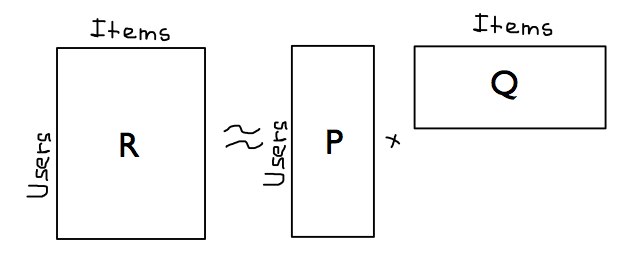## Singular Value Decomposition¶ Each matrix$X\in \mathbb{R}^{n \times d}$with rank$r$can be decomposed as $$X = U \Sigma V^\top ,$$ where •$U$- unitary matrix, which contains eigenvectors of$XX^\top$•$V$- unitary matrix, which contains eigenvectors of$X^\top X$•$\Sigma$- diagonal matrix with singular values$\sigma_i = \sqrt{\lambda_i}$## Truncated SVD¶ Truncated SVD with rank$K$$$X \approx \hat{X} = U_k \Sigma_k V_k^\top ,$$ where •$U_k$contains first$k$columns of$U$•$V_k$contains first$k$columns of$V$•$\Sigma_k$contains first$k$diagonal elements of$\Sigma$## SVD разложение¶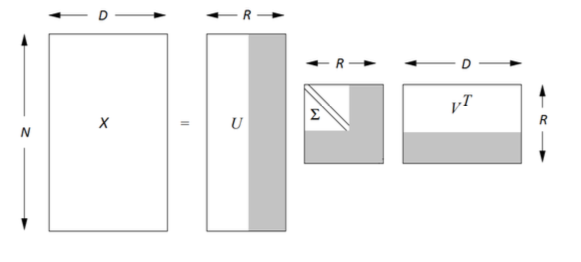It turns out that $$\hat{X} = \arg \min\limits_{A\in \mathbb{R}{n \times d}, rank(A) \leq k} \| X-A \|_{Frobenius}$$ ## Problems¶ • Have to fill missing values • with$0$• with baseline predictions • Optionally •$R' = R-B$and fill with$0$• In the end: •$P = U \Sigma^{1/2}$•$Q = \Sigma^{1/2}V^\top$•$\hat{R} = P^\top Q$• How do we calculate$p_u$for new user$u$given his rating vector$r_u$? ## Recalculation for new user¶ $$p_u = \arg \min\limits_p \|r_u - Vp \|^2 = \{\text{OLS solution}\} = \left( V^\top V \right)^{-1} V^\top r_u = V^\top r_u$$ •$p_u = V^\top r_u$is a vector of scalar products$[\langle v_1, r_u \rangle, \langle v_2, r_u \rangle, \dots, \langle v_k, r_u \rangle]$• Backward transformation from low-dimensional representation$p_u$to initial representation$\hat{r}_u$:$\hat{r}_u = Vp_u$## Latent Factor Model (Sparse SVD)¶ • Lets optimize $$\sum\limits_{u,i}(R_{ui} - \bar{R}_u - \bar{R}_i - \langle p_u, q_i \rangle)^2 \rightarrow \min\limits_{P, Q}$$ • Using SGD and going through pairs$(u,i)$with known$R_{ui}$: $$p_{uk} = p_{uk} + 2\alpha \left(q_{ik}(R_{ui} - \bar{R}_u - \bar{R}_i - \langle p_u, q_i \rangle\right)$$ $$q_{ik} = q_{ik} + 2\alpha \left(p_{uk}(R_{ui} - \bar{R}_u - \bar{R}_i - \langle p_u, q_i \rangle\right)$$ ## Latent Factor Model (Sparse SVD)¶ • Add regularization $$\sum\limits_{u,i}(R_{ui} - \bar{R}_u - \bar{R}_i - \langle p_u, q_i \rangle)^2 + \lambda \sum_u\| p_u \|^2 + \mu\sum_i\| q_i \|^2 \rightarrow \min\limits_{P, Q}$$ • Using SGD and going through pairs$(u,i)$with known$R_{ui}$: $$p_{uk} = p_{uk} + 2\alpha \left(q_{ik}(R_{ui} - \bar{R}_u - \bar{R}_i - \langle p_u, q_i \rangle) - \lambda p_{uk}\right)$$ $$q_{ik} = q_{ik} + 2\alpha \left(p_{uk}(R_{ui} - \bar{R}_u - \bar{R}_i - \langle p_u, q_i \rangle) - \mu q_{ik}\right)$$ # Factorization Machines¶ • Represent all users and items iteractions with one-hot encoded vector (of length$d=|U|+|I|$):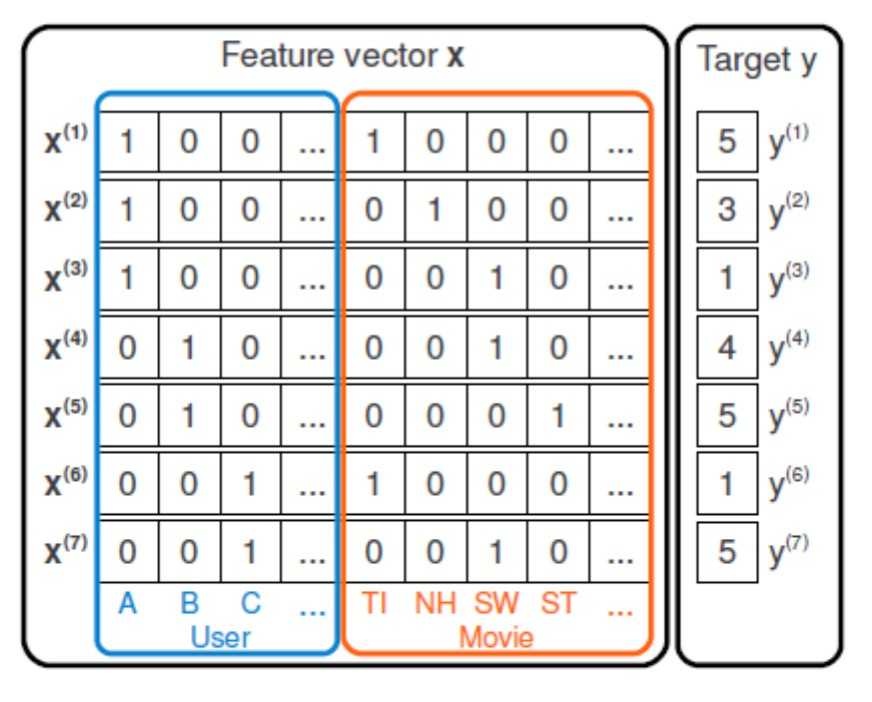• In such feature space we can consider various models: $$a(x) = w_0 + \sum\limits_{i=1}^d w_i x_i \quad \text(linear)$$$$a(x) = w_0 + \sum\limits_{i=1}^d w_i x_i + \sum\limits_{i=1,j=i+1}^d w_{ij} x_i x_j \quad \text(polynomial)$$ # Factorization Machines¶• Let's try to reduce the number of parameters and approximate$w_{ij}$with$\langle v_i, v_j\rangle$, where$v_i$is latent representation of feature$x_j$$$a(x) = w_0 + \sum\limits_{i=1}^d w_i x_i + \sum\limits_{i=1,j=i+1}^d \langle v_i, v_j\rangle x_i x_j \quad \text(fm)$$ # Factorization Machines¶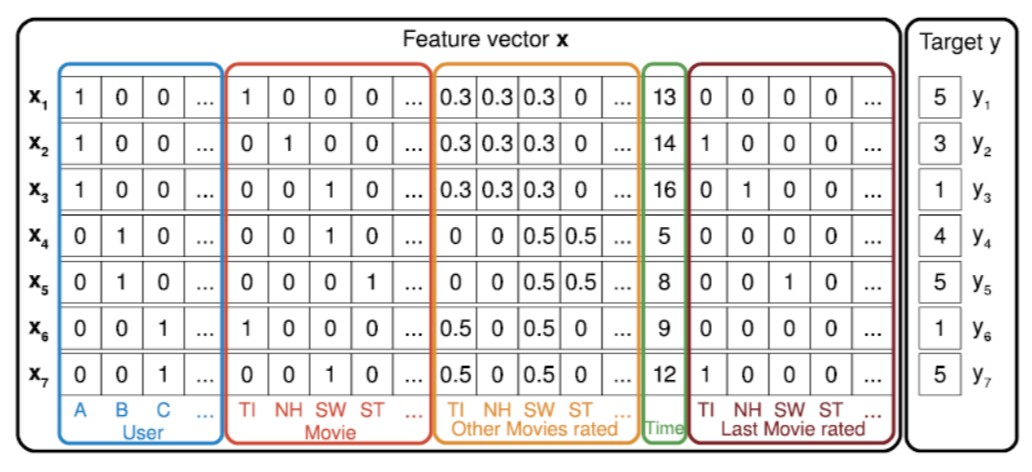# Classification-based models¶ • How can we adapt simple classification task to recommendation system domain? • Any problems here? # Measuring Quality¶ • Ratings accuracy • MAE, MSE • Event quality • F-score, ROC-AUC, PR-AUC • precision@k, recall@k • Ranking quality •$DCG@k(u) = \sum\limits_{p=1}^k \frac{rel(i,p)}{\log{(p+1)}}$•$nDCG@k(u) = \frac{DCG@k(u)}{\max{(DCG@k(u))}}\$
• What else is usefull to measure?